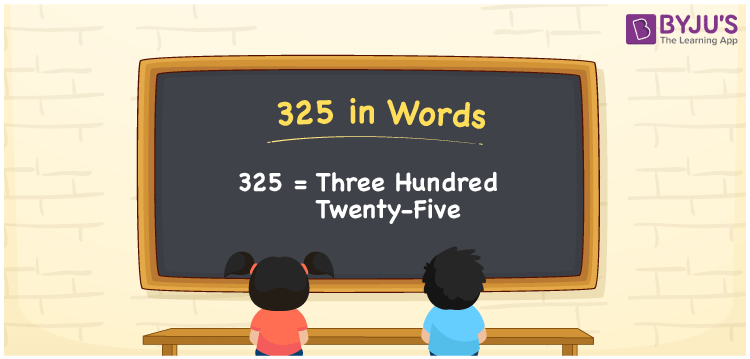# 325 in Words

325 in words can be written as Three Hundred Twenty-five. The concepts like counting numbers which are important from primary education are discussed here in brief. For instance, if you spend Rs. 325 in buying footwear, then you can say that “I bought footwear for Three Hundred Twenty-five Rupees”. The English alphabet is used to write 325 in words. Students can get a clear knowledge of numbers in words with the help of the study materials given at BYJU’S. The number 325 can be read as “Three Hundred Twenty-five” in English.

 325 in words Three Hundred Twenty-five Three Hundred Twenty-five in Numbers 325

## 325 in English Words## How to Write 325 in Words?

Place value chart and expanded form of 325 can be learnt easily in this section. There are three digits in the number 325. The place value chart for 325 is provided below for reference to help students understand the concepts effectively.

 Hundreds Tens Ones 3 2 5

The expanded form of 325 is provided below:

3 × Hundred + 2 × Ten + 5 × One

= 3 × 100 + 2 × 10 + 5 × 1

= 325

= Three Hundred Twenty-five

Hence, 325 in words is written as Three Hundred Twenty-five.

325 is a natural number that precedes 326 and succeeds 324.

325 in words – Three Hundred Twenty-five

Is 325 an odd number? – Yes

Is 325 an even number? – No

Is 325 a perfect square number? – No

Is 325 a perfect cube number? – No

Is 325 a prime number? – No

Is 325 a composite number? – Yes

## Frequently Asked Questions on 325 in Words

Q1

### How can 325 be written in words?

325 can be written as “Three Hundred Twenty-five” in words.
Q2

### Is 325 an odd number?

No, 325 is an odd number because it is not divisible by 2.
Q3

### Write Three Hundred Twenty-five in numbers.

Three Hundred Twenty-five can be written in numbers as 325.